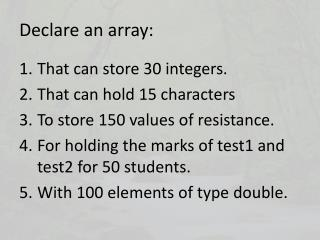DownloadDownload PresentationDeclare an array:

# Declare an array:

Télécharger la présentation## Declare an array:

- - - - - - - - - - - - - - - - - - - - - - - - - - - E N D - - - - - - - - - - - - - - - - - - - - - - - - - - -
##### Presentation Transcript

1. Declare an array: • That can store 30 integers. • That can hold 15 characters • To store 150 values of resistance. • For holding the marks of test1 and test2 for 50 students. • With 100 elements of type double.

2. Declare an array (ANSWER): • intaiExample ; • char acM ; • float afResistance ; • float marks   ; • double adWhat ;

3. Consider the following code segment: intaFolly = {5, 3, 2}; for (int x = 0; x < 6; x ++) { aFolly [x] = aFolly [x] + 3; } What is the value of aFolly ? What is the value of element five ? What is the value of the second element ? Write a statement to print the first and the fourth element.

4. Answer: When first declared: 5 3 2 0 0 0 After loop: 8 6 5 3 3 3 What is the value of aFolly ? 3 What is the value of element five ? 3 What is the value of the second element ? 6 Write a statement to print the first and the fourth element. printf (“ %d %d “, aFolly  , aFolly  );

5. Write C statements: • Declare a constant named MAX with value 15. • Declare an array of integers with MAX elements • Declare an array with 25 elements for storing wave lengths. • Display the third element of MyNumber. • Assign the value of 16.5 to the first and second elements of array XYZ

6. ANSWER: • constint MAX = 15; OR #define MAX 15 • IntaiTry [MAX]; • float afWaveLengths ; • printf ( “%d “ , MyNumber  ); • XYZ [ 0 ] = 16.5; XYZ  = 16.5;

7. Write C statements: • Access the fifth element of xArray. • Compare to see if the first element of aHM is more than 20. • Compare whether the element in b is the same as 55. • Add the value of the current element to the next element.

8. ANSWER: • xArray  ; • (aHM  > 20) • b == 55 • adC [ num + 1] + = adC [num]; OR adC [num + 1] = adC [num + 1] + adC [num];